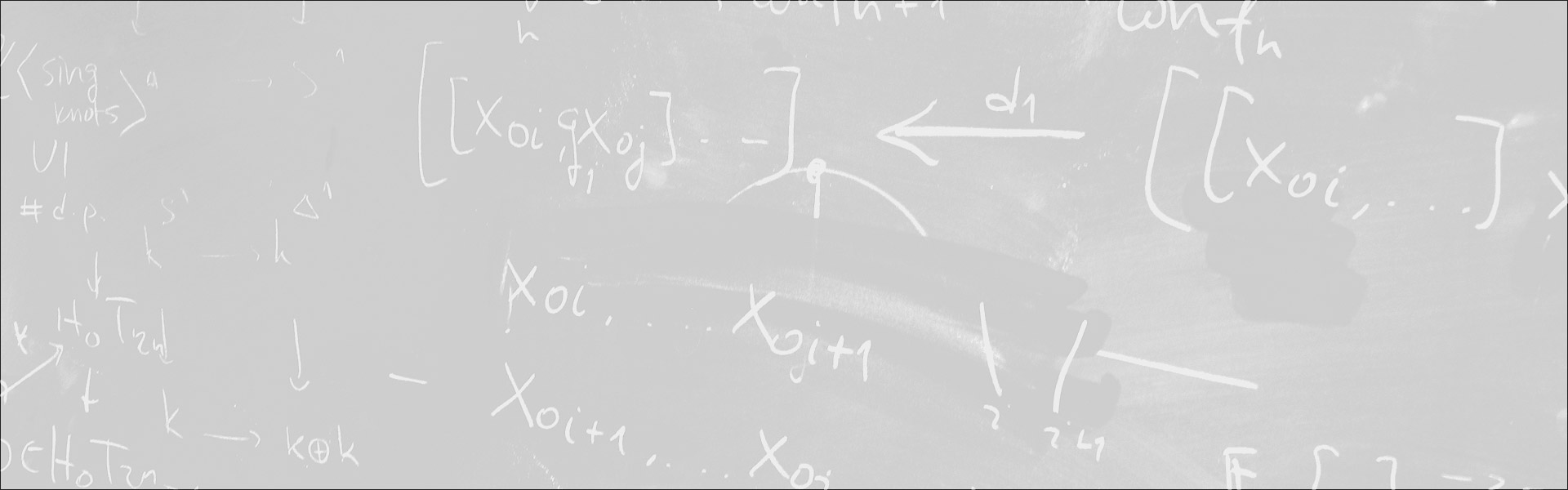Designed and built with care, filled with creative elements

Top# Quantitative Fundamental Theorem of Algebra

/  Évènements• Cet évènement est passé

## Quantitative Fundamental Theorem of Algebra

Using subresultants, we modify a recent real-algebraic proof due to Eisermann of the Fundamental Theorem of Algebra ([FTA]) to obtain the following quantitative information: in order to prove the [FTA] for polynomials of degree d, the Intermediate Value Theorem ([IVT]) is requested to hold for real polynomials of degree at most d^2. We also explain that the classical algebraic proof due to Laplace requires [IVT] for real polynomials of exponential degree. These quantitative results highlight the difference in nature of these two proofs.

- Séminaire Géométrie et théorie des modèles

#### Détails :

Orateur / Oratrice : Marie-Françoise Roy
Date : 31 janvier 2020
Horaire : 14h15 - 15h35
Lieu : ENS Salle W